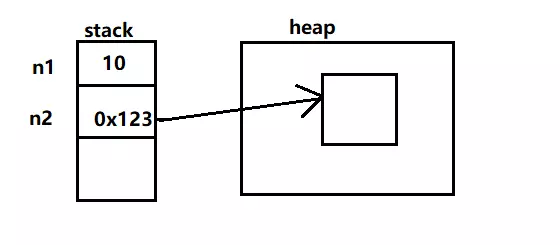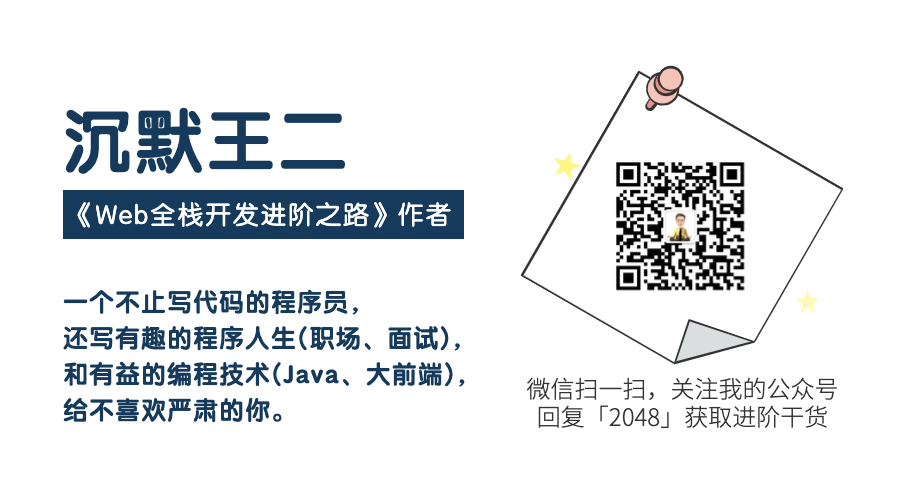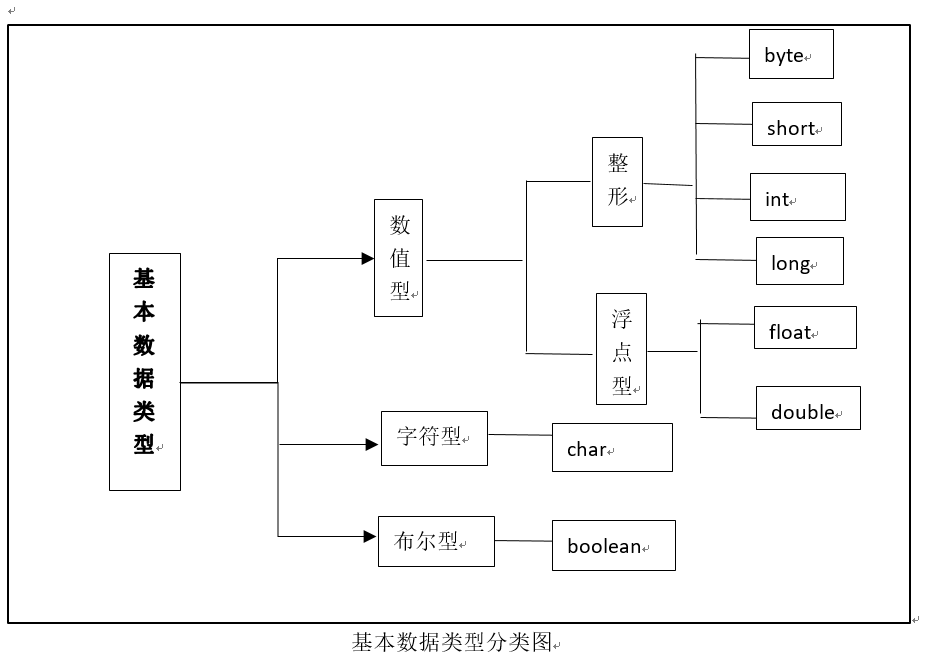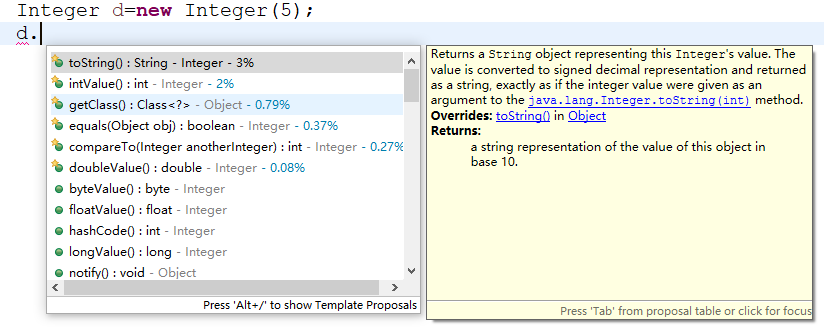• 1、基本和基本型封装型进行“==”运算符的比较基本型封装型将会自动拆箱变为基本型后再进行比较，因此Integer(0)会自动拆箱为int类型再进行比较，显然返回true；  int a = 220;  Integer b = 220;  System...

1、基本型和基本型封装型进行“==”运算符的比较，基本型封装型将会自动拆箱变为基本型后再进行比较，因此Integer(0)会自动拆箱为int类型再进行比较，显然返回true；

int a = 220;

Integer b = 220;

System.out.println(a==b);//true
2、两个Integer类型进行“==”比较， 如果其值在-128至127  ，那么返回true，否则返回false, 这跟Integer.valueOf()的缓冲对象有关，这里不进行赘述。

Integer c=3;

Integer h=3;

Integer e=321;

Integer f=321;

System.out.println(c==h);//true

System.out.println(e==f);//false

3、两个基本型的封装型进行equals()比较，首先equals()会比较类型，如果类型相同，则继续比较值，如果值也相同，返回true。

Integer a=1;

Integer b=2;

Integer c=3;

System.out.println(c.equals(a+b));//true

4、基本型封装类型调用equals(),但是参数是基本类型，这时候，先会进行自动装箱，基本型转换为其封装类型，再进行3中的比较。

int i=1;

int j = 2;

Integer c=3;

System.out.println(c.equals(i+j));//true

展开全文基本数据类型和包装类
• 基本类型包装类对象使用 == equals进行比较的结果 1、值不同，使用＝＝equals比较都返回false 2、值相同 使用＝＝比较基本类型基本类型基本类型－包装对象返回true 包装对象－包装对象返回...

基本类型的包装类对象使用 == 和 equals进行比较的结果

1、值不同，使用＝＝和equals比较都返回false

2、值相同

使用＝＝比较：

• 基本类型－基本类型、基本类型－包装对象返回true
• 包装对象－包装对象返回false
• 缓存中去的包装对象比较返回true（JVM 缓存部分基本类型常用的包装类对象，如 Integer 缓存 -128 ～ 127 ）
Integer i1 = 100;
Integer i2 = 100;
Integer i3 = 200;
Integer i4 = 200;

System.out.println(i1==i2); //打印true
System.out.println(i3==i4); //打印false

使用equals比较：

• 包装对象－基本类型返回true
• 包装对象－包装对象返回true

3、不同类型的对象对比，返回 false

JDK1.8，实验代码

byte b1 = 127;
Byte b2 = new Byte("127");
Byte b3 = new Byte("127");
System.out.println("Byte 基本类型和包装对象使用 == 比较 : " + (b1 == b2));
System.out.println("Byte 基本类型和包装对象使用 equals 比较 : " + b2.equals(b1));
System.out.println("Byte 包装对象和包装对象使用 == 比较 : " + (b2 == b3));
System.out.println("Byte 包装对象和包装对象使用 equals 比较 : " + b2.equals(b3));
System.out.println();

short s1 = 12;
Short s2 = new Short("12");
Short s3 = new Short("12");
System.out.println("Short 基本类型和包装对象使用 == 比较 : " + (s1 == s2));
System.out.println("Short 基本类型和包装对象使用 equals 比较 : " + s2.equals(s1));
System.out.println("Short 包装对象和包装对象使用 == 比较 : " + (s2 == s3));
System.out.println("Short 包装对象和包装对象使用 equals 比较 : " + s2.equals(s3));
System.out.println();

char c1 = 'A';
Character c2 = new Character('A');
Character c3 = new Character('A');
System.out.println("Character 基本类型和包装对象使用 == 比较 : " + (c1 == c2));
System.out.println("Character 基本类型和包装对象使用 equals 比较 : " + c2.equals(c1));
System.out.println("Character 包装对象和包装对象使用 == 比较 : " + (c2 == c3));
System.out.println("Character 包装对象和包装对象使用 equals 比较 : " + c2.equals(c3));
System.out.println();

int i1 = 10000;
Integer i2 = new Integer(10000);
Integer i3 = new Integer(10000);
System.out.println("Integer 基本类型和包装对象使用 == 比较 : " + (i1 == i2));
System.out.println("Integer 基本类型和包装对象使用 equals 比较 : " + i2.equals(i1));
System.out.println("Integer 包装对象和包装对象使用 == 比较 : " + (i2 == i3));
System.out.println("Integer 包装对象和包装对象使用 equals 比较 : " + i2.equals(i3));
System.out.println();

long l1 = 1000000000000000L;
Long l2 = new Long("1000000000000000");
Long l3 = new Long("1000000000000000");
System.out.println("Long 基本类型和包装对象使用 == 比较 : " + (l1 == l2));
System.out.println("Long 基本类型和包装对象使用 equals 比较 : " + l2.equals(l1));
System.out.println("Long 包装对象和包装对象使用 == 比较 : " + (l2 == l3));
System.out.println("Long 包装对象和包装对象使用 equals 比较 : " + l2.equals(l3));
System.out.println();

float f1 = 10000.111F;
Float f2 = new Float("10000.111");
Float f3 = new Float("10000.111");
System.out.println("Float 基本类型和包装对象使用 == 比较 : " + (f1 == f2));
System.out.println("Float 基本类型和包装对象使用 equals 比较 : " + f2.equals(f1));
System.out.println("Float 包装对象和包装对象使用 == 比较 : " + (f2 == f3));
System.out.println("Float 包装对象和包装对象使用 equals 比较 : " + f2.equals(f3));
System.out.println();

double d1 = 10000.111;
Double d2 = new Double("10000.111");
Double d3 = new Double("10000.111");
System.out.println("Double 基本类型和包装对象使用 == 比较 : " + (d1 == d2));
System.out.println("Double 基本类型和包装对象使用 equals 比较 : " + d2.equals(d1));
System.out.println("Double 包装对象和包装对象使用 == 比较 : " + (d2 == d3));
System.out.println("Double 包装对象和包装对象使用 equals 比较 : " + d2.equals(d3));
System.out.println();

boolean bl1 = true;
Boolean bl2 = new Boolean("true");
Boolean bl3 = new Boolean("true");
System.out.println("Boolean 基本类型和包装对象使用 == 比较 : " + (bl1 == bl2));
System.out.println("Boolean 基本类型和包装对象使用 equals 比较 : " + bl2.equals(bl1));
System.out.println("Boolean 包装对象和包装对象使用 == 比较 : " + (bl2 == bl3));
System.out.println("Boolean 包装对象和包装对象使用 equals 比较 : " + bl2.equals(bl3));

运行结果

Byte 基本类型和包装对象使用 == 比较 : true
Byte 基本类型和包装对象使用 equals 比较 : true
Byte 包装对象和包装对象使用 == 比较 : false
Byte 包装对象和包装对象使用 equals 比较 : true

Short 基本类型和包装对象使用 == 比较 : true
Short 基本类型和包装对象使用 equals 比较 : true
Short 包装对象和包装对象使用 == 比较 : false
Short 包装对象和包装对象使用 equals 比较 : true

Character 基本类型和包装对象使用 == 比较 : true
Character 基本类型和包装对象使用 equals 比较 : true
Character 包装对象和包装对象使用 == 比较 : false
Character 包装对象和包装对象使用 equals 比较 : true

Integer 基本类型和包装对象使用 == 比较 : true
Integer 基本类型和包装对象使用 equals 比较 : true
Integer 包装对象和包装对象使用 == 比较 : false
Integer 包装对象和包装对象使用 equals 比较 : true

Long 基本类型和包装对象使用 == 比较 : true
Long 基本类型和包装对象使用 equals 比较 : true
Long 包装对象和包装对象使用 == 比较 : false
Long 包装对象和包装对象使用 equals 比较 : true

Float 基本类型和包装对象使用 == 比较 : true
Float 基本类型和包装对象使用 equals 比较 : true
Float 包装对象和包装对象使用 == 比较 : false
Float 包装对象和包装对象使用 equals 比较 : true

Double 基本类型和包装对象使用 == 比较 : true
Double 基本类型和包装对象使用 equals 比较 : true
Double 包装对象和包装对象使用 == 比较 : false
Double 包装对象和包装对象使用 equals 比较 : true

Boolean 基本类型和包装对象使用 == 比较 : true
Boolean 基本类型和包装对象使用 equals 比较 : true
Boolean 包装对象和包装对象使用 == 比较 : false
Boolean 包装对象和包装对象使用 equals 比较 : true

ps：可以延伸一个问题，基本类型与包装对象的拆/装箱的过程

【Java面试题与答案】整理推荐

展开全文• Java 的每个基本类型都对应了一个包装类型，比如说 int 的包装类型为 Integer，double 的包装类型为 Double。基本类型和包装类型的区别主要有以下 4 点。

六年前，我从苏州回到洛阳，抱着一幅“海归”的心态，投了不少简历，也“约谈”了不少面试官，但仅有两三个令我感到满意。其中有一位叫老马，至今还活在我的手机通讯录里。他当时扔了一个面试题把我砸懵了：说说基本类型和包装类型的区别吧。

我当时二十三岁，正值青春年华，从事 Java 编程已有 N 年经验（N < 4），自认为所有的面试题都能对答如流，结果没想到啊，被“刁难”了——原来洛阳这块互联网的荒漠也有技术专家啊。现在回想起来，脸上不自觉地泛起了羞愧的红晕：主要是自己当时太菜了。不管怎么说，是时候写篇文章剖析一下基本类型和包装类型的区别了。

Java 的每个基本类型都对应了一个包装类型，比如说 int 的包装类型为 Integer，double 的包装类型为 Double。基本类型和包装类型的区别主要有以下 4 点。

01、包装类型可以为 null，而基本类型不可以

别小看这一点区别，它使得包装类型可以应用于 POJO 中，而基本类型则不行。

POJO 是什么呢？这里稍微说明一下。

POJO 的英文全称是 Plain Ordinary Java Object，翻译一下就是，简单无规则的 Java 对象，只有属性字段以及 setter 和 getter 方法，示例如下。

class Writer {
private Integer age;
private String name;

public Integer getAge() {
return age;
}

public void setAge(Integer age) {
this.age = age;
}

public String getName() {
return name;
}

public void setName(String name) {
this.name = name;
}
}

和 POJO 类似的，还有数据传输对象 DTO（Data Transfer Object，泛指用于展示层与服务层之间的数据传输对象）、视图对象 VO（View Object，把某个页面的数据封装起来）、持久化对象 PO（Persistant Object，可以看成是与数据库中的表映射的 Java 对象）。

那为什么 POJO 的属性必须要用包装类型呢？

《阿里巴巴 Java 开发手册》上有详细的说明，我们来大声朗读一下（预备，起）。

数据库的查询结果可能是 null，如果使用基本类型的话，因为要自动拆箱（将包装类型转为基本类型，比如说把 Integer 对象转换成 int 值），就会抛出 NullPointerException 的异常。

02、包装类型可用于泛型，而基本类型不可以

泛型不能使用基本类型，因为使用基本类型时会编译出错。

List<int> list = new ArrayList<>(); // 提示 Syntax error, insert "Dimensions" to complete ReferenceType
List<Integer> list = new ArrayList<>();

为什么呢？因为泛型在编译时会进行类型擦除，最后只保留原始类型，而原始类型只能是 Object 类及其子类——基本类型是个特例。

03、基本类型比包装类型更高效

基本类型在栈中直接存储的具体数值，而包装类型则存储的是堆中的引用。很显然，相比较于基本类型而言，包装类型需要占用更多的内存空间。假如没有基本类型的话，对于数值这类经常使用到的数据来说，每次都要通过 new 一个包装类型就显得非常笨重。

03、两个包装类型的值可以相同，但却不相等

两个包装类型的值可以相同，但却不相等——这句话怎么理解呢？来看一段代码就明明白白了。

Integer chenmo = new Integer(10);
Integer wanger = new Integer(10);

System.out.println(chenmo == wanger); // false
System.out.println(chenmo.equals(wanger )); // true

两个包装类型在使用“”进行判断的时候，判断的是其指向的地址是否相等。chenmo 和 wanger 两个变量使用了 new 关键字，导致它们在“”的时候输出了 false。

chenmo.equals(wanger) 的输出结果为 true，是因为 equals 方法内部比较的是两个 int 值是否相等。源码如下。

private final int value;

public int intValue() {
return value;
}
public boolean equals(Object obj) {
if (obj instanceof Integer) {
return value == ((Integer)obj).intValue();
}
return false;
}

瞧，虽然 chenmo 和 wanger 的值都是 10，但他们并不相等。换句话说就是：将“==”操作符应用于包装类型比较的时候，其结果很可能会和预期的不符

04、自动装箱和自动拆箱

既然有了基本类型和包装类型，肯定有些时候要在它们之间进行转换。把基本类型转换成包装类型的过程叫做装箱（boxing）。反之，把包装类型转换成基本类型的过程叫做拆箱（unboxing）。

在 Java SE5 之前，开发人员要手动进行装拆箱，比如说：

Integer chenmo = new Integer(10);  // 手动装箱
int wanger = chenmo.intValue();  // 手动拆箱

Java SE5 为了减少开发人员的工作，提供了自动装箱与自动拆箱的功能。

Integer chenmo  = 10;  // 自动装箱
int wanger = chenmo;     // 自动拆箱

Integer chenmo = Integer.valueOf(10);
int wanger = chenmo.intValue();

也就是说，自动装箱是通过 Integer.valueOf() 完成的；自动拆箱是通过 Integer.intValue() 完成的。理解了原理之后，我们再来看一道老马当年给我出的面试题。

// 1）基本类型和包装类型
int a = 100;
Integer b = 100;
System.out.println(a == b);

// 2）两个包装类型
Integer c = 100;
Integer d = 100;
System.out.println(c == d);

// 3）
c = 200;
d = 200;
System.out.println(c == d);

答案是什么呢？有举手要回答的吗？答对的奖励一朵小红花哦。

第一段代码，基本类型和包装类型进行 == 比较，这时候 b 会自动拆箱，直接和 a 比较值，所以结果为 true。

第二段代码，两个包装类型都被赋值为了 100，这时候会进行自动装箱，那 == 的结果会是什么呢？

我们之前的结论是：将“==”操作符应用于包装类型比较的时候，其结果很可能会和预期的不符。那结果是 false？但这次的结果却是 true，是不是感觉很意外？

第三段代码，两个包装类型重新被赋值为了 200，这时候仍然会进行自动装箱，那 == 的结果会是什么呢？

吃了第二段代码的亏后，是不是有点怀疑人生了，这次结果是 true 还是 false 呢？扔个硬币吧，哈哈。我先告诉你结果吧，false。

为什么？为什么？为什么呢？

事情到了这一步，必须使出杀手锏了——分析源码吧。

之前我们已经知道了，自动装箱是通过 Integer.valueOf() 完成的，那我们就来看看这个方法的源码吧。

public static Integer valueOf(int i) {
if (i >= IntegerCache.low && i <= IntegerCache.high)
return IntegerCache.cache[i + (-IntegerCache.low)];
return new Integer(i);
}

难不成是 IntegerCache 在作怪？你猜对了！

private static class IntegerCache {
static final int low = -128;
static final int high;
static final Integer cache[];

static {
// high value may be configured by property
int h = 127;
int i = parseInt(integerCacheHighPropValue);
i = Math.max(i, 127);
h = Math.min(i, Integer.MAX_VALUE - (-low) -1);
high = h;

cache = new Integer[(high - low) + 1];
int j = low;
for(int k = 0; k < cache.length; k++)
cache[k] = new Integer(j++);

// range [-128, 127] must be interned (JLS7 5.1.7)
assert IntegerCache.high >= 127;
}
}

大致瞟一下这段代码你就全明白了。-128 到 127 之间的数会从 IntegerCache 中取，然后比较，所以第二段代码（100 在这个范围之内）的结果是 true，而第三段代码（200 不在这个范围之内，所以 new 出来了两个 Integer 对象）的结果是 false。

看完上面的分析之后，我希望大家记住一点：当需要进行自动装箱时，如果数字在 -128 至 127 之间时，会直接使用缓存中的对象，而不是重新创建一个对象

自动装拆箱是一个很好的功能，大大节省了我们开发人员的精力，但也会引发一些麻烦，比如下面这段代码，性能就很差。

long t1 = System.currentTimeMillis();
Long sum = 0L;
for (int i = 0; i < Integer.MAX_VALUE;i++) {
sum += i;
}
long t2 = System.currentTimeMillis();
System.out.println(t2-t1);

sum 由于被声明成了包装类型 Long 而不是基本类型 long，所以 sum += i 进行了大量的拆装箱操作（sum 先拆箱和 i 相加，然后再装箱赋值给 sum），导致这段代码运行完花费的时间足足有 2986 毫秒；如果把 sum 换成基本类型 long，时间就仅有 554 毫秒，完全不一个等量级啊。

05、最后

谢谢大家的阅读，原创不易，喜欢就点个赞，这将是我最强的写作动力。如果你觉得文章对你有所帮助，也蛮有趣的，就关注一下我的公众号，谢谢。展开全文Java 面试官 基本类型 包装类型
• 最近刷题的时候碰到了不少基本数据类型和包装类进行比较的问题，一开始做的有点蒙，错多了之后就自己整理了一下，特记录下来加深印象。 在java中不能定义基本数据类型对象，为了能将基本数据类型视为对象处理，...

最近刷题的时候碰到了不少基本数据类型和包装类进行比较的问题，一开始做的有点蒙，错多了之后就自己整理了一下，特记录下来加深印象。

在java中不能定义基本数据类型对象，为了能将基本数据类型视为对象处理，并且能够调用方法简化其使用过程，java为每个基本类型都提供了包装类。
下面是8种基本数据类型图解下面是基本数据类型及其包装类对照表：

基本数据类型包装类
intInteger
byteByte
shortShort
longLong
floatFloat
doubleDouble
charChar
booleanBoolean

包装类提供了多种常用方法，如下图：其中equals方法在本篇中有所涉及，摘录其源码如下：

public boolean equals(Object obj) {
if (obj instanceof Integer) {
return value == ((Integer)obj).intValue();
}
return false;
}

可以看出，先通过instanceof判断比较对象obj是否是Integer的类实例，如果不是，则return false，若是，则强制转换为Integer类并通过intValue（）方法取值，比较调用方法的对象的值和该值是否相等，返回比较结果。
包装类往往涉及到比较两个对象或者比较对象和基本数据类型的大小的问题。
下面以最常用的Integer类为例，用程序说明几种判断方式。
通过总结得到以下四点：
1.同样被new出的两个相同类型的对象一定是不“==”的，因为==比较的是位置，而new出的对象一定是全新地址的，但是用equals比较时是比较的数值，是相等的。

Integer a=new Integer(5);
Integer b=new Integer(5);
System.out.println(a==b);//return false
System.out.println(a.equals(b));//return true

2.在“==”进行比较时，有以下原则：
1）不用new得到新对象，当要赋的值在区间[-128，127]时，该值会被缓存到IntegerCache.cache中，此时赋值的是同一对象，用“==”比较会返回ture；

Integer a=5;
Integer b=5;
System.out.println(a==b);//return true

2）当要赋的值在(-∞，-128）或（127，+∞）时，即时不用new得到新对象，用“==”比较也会返回false；

Integer a=128;
Integer b=128;
Integer c=-129;
Integer d=-129;
System.out.println(a==b);//return false
System.out.println(c==d);//return false

3）当用new赋值时，一定返回false；
3.当Integer对象与基本类型做比较时，默认将Integer对象做拆箱操作，比较基本类型，而基本类型的比较只与数值有关，因此数值一样就返回true；

Integer a=new Integer(5);
int c=5;
System.out.println(a==c);//return true

4.若两个Byte对象相加，则会自动升格为int，只能赋值给int或更高精度的类型，因此赋值给Byte一定出错。

Byte a = new Byte((byte) 5);
Byte b = new Byte((byte) 5);
Byte c = a + b;
/*
* 报错如下：
* Type mismatch: cannot convert from int to Byte
*/

希望有缺漏的地方各位能够予以补充。

展开全文java 基本数据类型 比较大小
• 包装类和基本类型

万次阅读 多人点赞 2019-03-06 09:18:53
什么是包装类 所谓包装类，就是能够直接将简单类型的变量表示为一个类，在执行变量类型的相互转换时，我们会大量使用这些包装类包装类 包装类有以下用途 1.集合不允许存放基本数据类型，故常用包装类 2.包含了每...
• 基本数据类型的 优点: 1.计算的效率高 2.不会由于常量池引起比较大小错误(比较数据更准确) 缺点 1.当数据库查询出结果封装到结果集时,如何返回的值为null时,会将结果赋值给字段,运行时会报错,不能将null值赋值...基本数据类型
• 在Java初学阶段，想必很多人都会先学Java中的一些基本类型以及他们的包装类,如下 int --> Integer long --> Long double --> Double ...等等 很多人在学的时候感觉这用处不大啊，也不知道什么时候用，该...java包装类
• 基本类型和包装类的区别

万次阅读 2018-03-24 16:02:52
区别： 1.基本类型存储在栈里，包装类型存储在堆里。因为栈的效率更高，所以保留了基本类型。 2.包装类是对象，拥有方法字段，对象的调用是引用对象的地址。... 基本类型包装类 以及其默认值,包装...基本类型 默认值
• 基本类型和包装类之间的转换基本类型和包装类之间经常需要互相转换，以 Integer 为例（其他几个包装类的操作雷同哦）：在 JDK1.5 引入自动装箱和拆箱的机制后，包装类和基本类型之间的转换就更加轻松便利了。...
•java
• 基本类型和包装类型的区别详解

千次阅读 多人点赞 2019-11-30 22:20:01
他当时扔了一个面试题把我砸懵了：说说基本类型和包装类型的区别吧。 我当时二十三岁，正值青春年华，从事 Java 编程已有 N 年经验（N < 4），自认为所有的面试题都能对答如流，结果没想到啊，被“刁难”了——...基本类型
• **1、基本和基本型封装型进行“==”运算符的比较基本型封装型将会自动拆箱变为基本型后再进行比较，因此Integer(0)会自动拆箱为int类型再进行比较，如1,4行，显然返回true。 2、两个Integer类型进行“==”比较...equals
• Java 基本类型包装类

千次阅读 2021-03-08 05:56:26
Java基本类型包装类：基本数据类型 基本类型包装类byte Byteshort Shortint Integerlong Longfloat Floatdouble Doublechar Charac...
• 基本类型转换为字符串有三种方法： 使用包装类的 toString() 方法 使用String类的 valueOf() 方法 用一个空字符串加上基本类型，得到的就是基本类型数据对应的字符串 //将基本类型转换为字符public class Test{ ...
• 接下来我们也顺便看下基本类型包装类和起对应的对象池 JDK1.8 八大基本类型 Java基本类型共有八种，基本类型可以分为三类， 字符类型char 布尔类型boolean 数值类型byte、short、int、long、float、double ...基本类型的包装类 对象池
• 1、包装类是对象，拥有方法字段，对象的调用都是通过引用对象的地址；基本类型不是2、包装类型是引用的传递；基本类型是值的传递3、声明方式不同： 基本数据类型不需要new关键字； 包装类型需要new在堆内存中进行...
• 基本数据类型没有equals方法intInteger比较，Integer会自动拆箱，== equals都肯定为trueintnew Integer比较，Integer会自动拆箱，调用intValue方法, 所以 == equals都肯定为trueIntegerInteger比较的...
• java八种基本数据类型及包装类详解

万次阅读 多人点赞 2018-12-20 10:14:40
详解一、八种基本数据类型常识 1.1、基本常识表 对于上图有以下几点需要注意： java八种基本数据类型分为四类八种，四类分别为整型、浮点型、布尔型、字符型；八种分别为byte、short、int、long、float、double...java 基本数据类型 面试题
• 如图所见，我们在定义（八种基本数据类型）Int a 时，引用（地址）在栈空间，内容在常量池。a通过地址找到其内容。而Integer（引用类型）定义的东西也在栈空间，但是其内容在堆空间。 先看看下面的几道题目 do....
• java数据类型包括两大基本数据类型和引用数据类型。 引用数据类型：、接口、数组。 基本数据类型：数值型{（整数类型：byte、short、int、long）（浮点类型：float、double）}、字符型（char）、布尔型...
• 在初学JAVA时我们接触到的都是一些基本的数据类型，比如int , float,double等。但是对于高等语言JAVA等面向对象的语言来说，一切都是对象。如此看来这些基本数据类型显得就有些‘单薄’--------它只有... (1)包装类和
• Java 中基本类型和包装类之间的转换 基本类型和包装类之间经常需要互相转换，以 Integer 为例（其他几个包装类的操作雷同哦）： 在 JDK1.5 引入自动装箱和拆箱的机制后，包装类和基本类型之间的转换...java 基本类型
• 基本类型和字符串的相互转换 基本类型转字符串： 最常用： 直接把这个数字加上空字符串 1. 12 + "" 其他方法：不常用 2. 使用String的静态方法valueOf进行转换 3. 调用其包装类的toString方法。 ...
• 问题：两者都为引用类型，为什么第一种情况能得到修改过后的值，而第二种不能？ // 案例1 Customer customer = new Customer(); customer.setCust_name("aaa"); List&lt;Customer&gt; ...八种包装类 引用类型
• Java 中有 8 个基本类型，分别对应的包装类如下 byte -- Byte boolean -- Boolean short -- Short char -- Character int -- Integer long -- Long float -- Float double -- Double 为什么要有包装类 ...
• Java中的8种基本数据类型都有对应的对象类型，但是既然有了基本数据类型，包装类型有什么作用呢？它们有什么区别呢？ 答案是包装类型的出现是为了方便对基本类型进行操作。包装类型可以解决一些基本...2.基本类型和...包装类型 基本数据类型 Integer 自动装箱
• eg 基本类型默认为有值的 用包装类不传值得是否默认为空 mybatisplus进行操作时更容易进行是否非空的判断java...